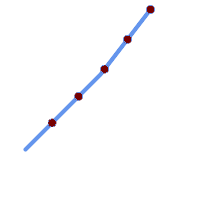## Name

ST_LineInterpolatePoints — Returns points interpolated along a line at a fractional interval.

## Synopsis

`geometry ST_LineInterpolatePoints(`geometry a_linestring, float8 a_fraction, boolean repeat`)`;

## Description

Returns one or more points interpolated along a line at a fractional interval. The first argument must be a LINESTRING. The second argument is a float8 between 0 and 1 representing the spacing between the points as a fraction of line length. If the third argument is false, at most one point will be constructed (the function will be equivalent to ST_LineInterpolatePoint.)

If the result has zero or one points, it will be returned as a POINT. If it has two or more points, it will be returned as a MULTIPOINT.

Availability: 2.5.0This function supports 3d and will not drop the z-index.This function supports M coordinates.

## ExamplesA LineString with the interpolated points every 20%

```--Return points each 20% along a 2D line
SELECT ST_AsText(ST_LineInterpolatePoints('LINESTRING(25 50, 100 125, 150 190)', 0.20))
st_astext
----------------
MULTIPOINT(51.5974135047432 76.5974135047432,78.1948270094864 103.194827009486,104.132163186446 130.37181214238,127.066081593223 160.18590607119,150 190)
```